V.6 No 1 57 The problem of physical time in today physicsTo see the cause of this difference, determine the speeds of wave propagation for the first and second approaches to this problem. It will be sufficient to take derivatives in time of the systems (3.3) and (3.8) respectively. In the case of moving frame yield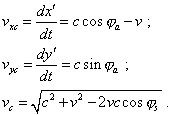(3.13)
 In the case of moving source yield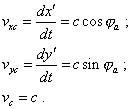(3.14)
 Thus we see, if the frame moved with respect to source, the speed of light in the moving frame varies from c + v to c - v , and in the second case, when the source moved with respect to the frame, the speed of light is isotropic and equal to c. This is because the frame rests with respect to medium in which the wave processes propagate. It appears that when we did the transformation from one frame to another, premising the isotropic propagation of light in the resting frame, we silently premised that the resting frame relates to the medium in which the light waves propagate. But when we considered processes immediately in the moving frame taking it as the resting, then despite our additional claims of its motion, it became resting by the meaning which we gave it – with respect to the luminiferous medium, irrespectively of our additional claims. This duality raises a question: should we account some additional features of the frame, could we yield the results of first modelling in limits of second? It appears, we could not, as the difference arises just when we rise the question, whether the frame moves with respect to the luminiferous medium. Answering this question, we have to remember of this medium, to interrelate the frame with it, to determine the speed of moving frame and of the source with respect to this medium and only after it we may solve the problem in the moving frame. The very fact of source’s motion in relation to the given frame does not evidence that the source is at rest with respect to the aether. If both the frame and source move with some speeds with respect to the luminiferous medium, the solution will depend on this co-motion. To show it, premise that the source moves with respect to the luminiferous medium with the speed vs  with the positive direction of the axis x of the frame related to this medium. The second inertial frame moves in the same direction with the speed vr . We should immediately draw our attention: as opposite to previous models, the frame and source move in positive direction. We made this change to provide the common approach, supposing that the speed of frame can be both positive and negative. In this way we on one hand generalise our model, and on the other keep the possibility to compare our results with the models studied before. To plot the pattern, how the equiphase surfaces propagate in the moving frame, we have to apply sequentially the results of previous particular models. Let us first determine the shape of some arbitrarily chosen equiphase surface in relation to the resting frame. According to (3.8), noting the signs, its equation will be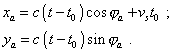(3.15)
 Now, knowing the pattern of equiphase surfaces in the resting frame, let us pass to the moving frame, applying the transformation (3.2). Yield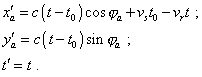(3.16)
 As we expected, this solution is the superposition of two above models. The dynamic diagram plotted with (3.16) is shown in Fig. 3.4.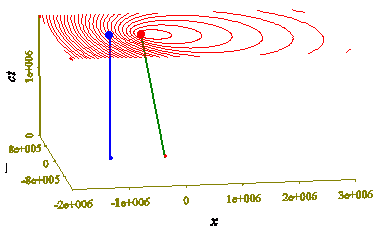Fig. 3.4. Dynamic diagram of light equiphase surfaces propagation when the moving source and moving frame with the speeds vs = - 0,8 c and vr = - 0,6 c  relatively, for the moments of time from t0 = 0 to t0 = 6T , where t0   is the moment of radiation of the equiphase surface; the world line of the observer is denoted in blue
 We see from the construction that the pattern of transformation of equiphase surfaces did not change comparing with Fig. 3.2  which, as we revealed, was calculated from the point of frame resting with respect to the luminiferous medium. Only the inclination of the source’s world line has changed. Hence, the transformation of equiphase surfaces is absolute and independent of the frame. At the same time it does not mean that the observer in the moving frame will yield unchanged characteristics of the light signal irrespectively of the speed of its frame with respect to the luminiferous medium. To show it, determine the propagation speed of equiphase surface from the point of observer in the moving frame. Use the above technique and differentiate the system (3.16) in time. We will yield in full accordance with (3.13)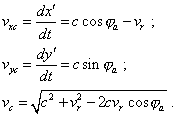(3.17)
 This expression corroborates again that, when we took the moving frame as resting, then, taking vr = 0 , we made it resting not only with respect to the observer but with respect to the luminiferous medium, so we automatically yielded the isotropic propagation of light, in accordance with (3.14) and (3.17). Now determine the regularity of transformation of the period between the equiphase surfaces from the point of moving observer. Noting the above technique, suppose that the source radiates the equiphase surface at some moment t0 and the observer detects it at the point x'1 of the moving frame at the moment t1 . Then, in accordance with (3.16),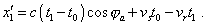(3.18)
 Suppose that the next pulse radiated by the source at t0 + T   the observer detects at t1 + T '  at the same point of the moving frame. For this next pulse yield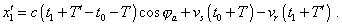(3.19)
 Subtracting (3.18) from (3.19), with a simple transformation, yield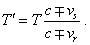(3.20)